# Moore circuit timing diagram

• Engineering
• fiksx

## Homework Statement

Fig. 3-3 is a Moore type sequential circuit composed of a positive edge triggered D flip-flop and a combinational circuit. X is input, Y is an output, and CLK is a clock. Please show how Qo, Q1, and Y change when input X is given to this circuit at the timing diagram shown in Figure 3-4.

I got the answer is y=0 but in timing diagram it is not zero why?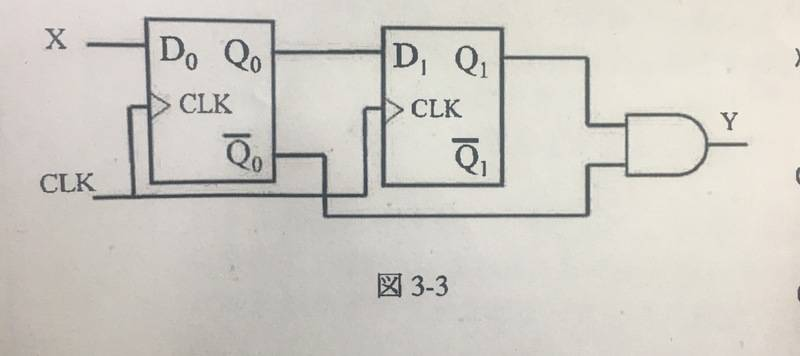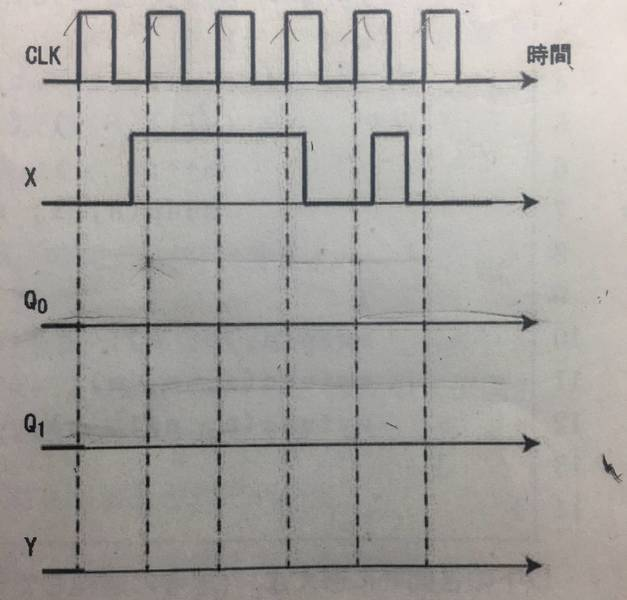my attempt to draw timing diagram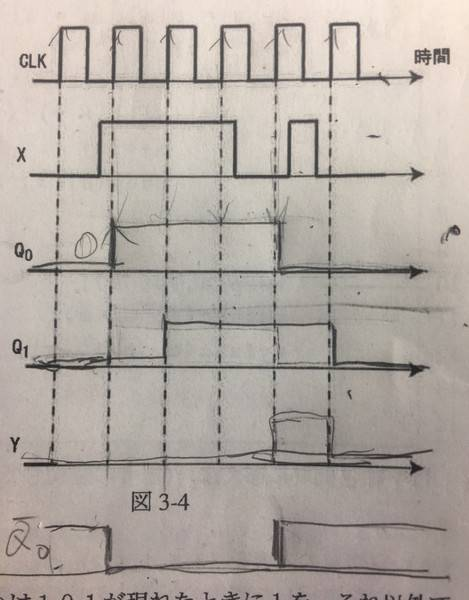Why y is not zero in timing diagram?

Figure 3-5 shows the state transition diagram of Moore type sequential circuit M that observes the time series of input X and outputs 1 when time series 010 or 101 appears, and 0 otherwise (Incomplete). Let S0 be the initial state (assume that 0 is input consecutively twice or more consecutively) and complete this state transition diagram according to the notation without increasing the number of states. Note that the time series will continue indefinitely.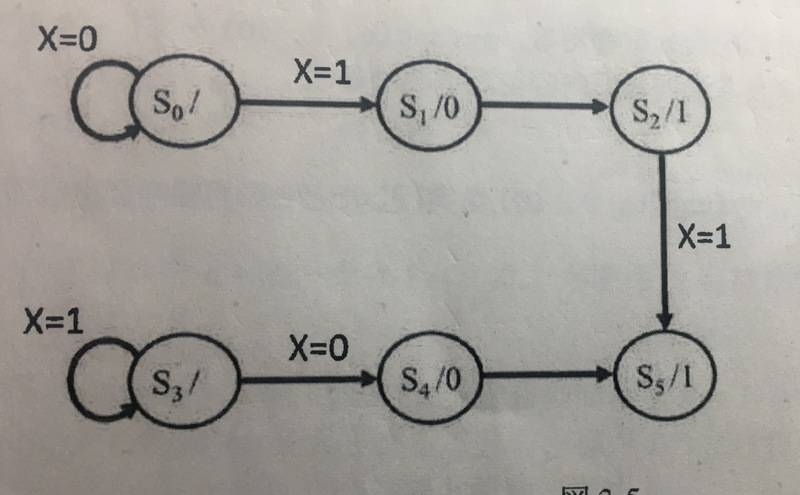Here i really don't understand what the question want?
Should i make 010/101 from the diagram?
Can someone give me hint?
Thankyou!

## The Attempt at a Solution

#### Attachments

Why y is not zero in timing diagram?
Why do you think it should be? What are the levels of the inputs to the AND gate during the 5th clock cycle?

Why do you think it should be? What are the levels of the inputs to the AND gate during the 5th clock cycle?
it is q1=x and ##\bar{q_0}##=##\bar{x}##. then ##y=\bar{x}.x=0## right?
what do you mean level input?
in the 5th clock cycle y produces 1?

it is q1=x and ¯^q0q0^¯\bar{\hat{q_0}}=¯^x
Don't punish yourself (and others) with unnecessary notation. Use /Q0 or ~Q0 or Q0' to indicate the "Q-not" signal.

what do you mean level input?
I mean logic level. Either a 1 or 0.

in the 5th clock cycle it produces 1?
Your attempt to draw the timing diagram shows Y being a "1" and a "0" at the same time, for the 5th clock cycle. If you are saying that it should be a "0", you are incorrect. I ask again: what are the logic levels at the inputs of the AND gate for the 5th clock cycle?

Don't punish yourself (and others) with unnecessary notation. Use /Q0 or ~Q0 or Q0' to indicate the "Q-not" signal.

I mean logic level. Either a 1 or 0.

Your attempt to draw the timing diagram shows Y being a "1" and a "0" at the same time, for the 5th clock cycle. If you are saying that it should be a "0", you are incorrect. I ask again: what are the logic levels at the inputs of the AND gate for the 5th clock cycle?

it is 1?
as you can see in my picture, ~Q0 =1 and q1=1 right?

~Q0 =1 and q1=1 right?
In clock cycle 5, you are correct. So based on that, for clock cycle 5, what logic level do you think Y should be?

By the way, be consistent in your notation. Rather than "~Q0 =1 and q1=1", which mixes boldface and capitalization, better "~Q0 =1 and Q0=1" or "~Q0 =1 and Q1=1". Whatever you prefer.

In clock cycle 5, you are correct. So based on that, for clock cycle 5, what logic level do you think Y should be?

By the way, be consistent in your notation. Rather than "~Q0 =1 and q1=1", which mixes boldface and capitalization, better "~Q0 =1 and Q0=1" or "~Q0 =1 and Q1=1". Whatever you prefer.
y is 1 ,but why when i count ##\bar{q_0}##=##\bar{x}##. then ##y=\bar{x}.x=0## it produce y always zero?

Sorry, I am having trouble understanding what you are asking. I say I'm "sorry" because I try to adhere to the Farsi expression: "the listener has the obligation to understand what the speaker is saying". Or something like that. In this case, I am failing. Can you explain what you are asking another way? Or maybe graphically?

Sorry, I am having trouble understanding what you are asking. I say I'm "sorry" because I try to adhere to the Farsi expression: "the listener has the obligation to understand what the speaker is saying". Or something like that. In this case, I am failing. Can you explain what you are asking another way? Or maybe graphically?
y is 1 in 5th clock cycle
when i count ##\bar{q_0}##=##\bar{x}##. then ##y=\bar{x}.x=0## it produce ##y=0##, it means y is always zero, why is it different from the one i got in timing diagram? the timing diagram produce all y=0 except in 5th cycle. i want to know why is that happen

y is 1 in 5th clock cycle
when i count ##\bar{q_0}##=##\bar{x}##. then ##y=\bar{x}.x=0## it produce ##y=0##, it means y is always zero, why is it different from the one i got in timing diagram? the timing diagram produce y=1 in 5th clock cycle, it should be all y always zero isn't it?
i want to know why is that happen

If what you are saying is that the Q1 input to the AND gate is based on X and the /Q0 input to the AND gate is based on /X, and therefore X AND /X must be 0, then your error is in treating this as a combinational logic circuit. It is not. The sequential (clocked) nature of the circuit must always be considered.

•SunThief
If what you are saying is that the Q1 input to the AND gate is based on X and the /Q0 input to the AND gate is based on /X, and therefore X AND /X must be 0, then your error is in treating this as a combinational logic circuit. It is not. The sequential (clocked) nature of the circuit must always be considered.

ok so you mean, there is no relation between the timing diagram and the output?

No I am not saying that. The timing diagram is closely related (edit: exactly related) to the sequential circuit (which usually has an output). For a given sequential circuit, the timing diagram is used to determine the output. Sometimes you will be given a timing diagram and expected to generate a sequential circuit.

Last edited:
No I am not saying that. The timing diagram is closely related to the sequential circuit (which usually has an output). For a given sequential circuit, the timing diagram is used to determine the output. Sometimes you will be given a timing diagram and expected to generate a sequential circuit.
but why ##y## logic level in 5th clock cycle is 1? while it should be all 0?
is the timing diagram that i drew correct?

Hi. Been trying to follow this offline. Not sure that I do :-), but I'll jump in. The OP seems to keep trying to relate the output y to x'. If I'm understanding this correctly, I think lewando's key point...

The sequential (clocked) nature of the circuit must always be considered.

... is that the output is based on a sequential process that precedes it. So the focus would be on what inputs are present at the final AND gate for the clock iteration you're trying to assess.

... follow up to above, so this isn't true:

x=0 it produce y=0 it means y is always zero

why y logic level in 5th clock cycle is 1?
You already agreed that this was the case (in posts 5, 7 and 9). In the 5th clock cycle, both inputs to the AND gate are at logic level 1. So why is this not clear?

You already agreed that this was the case (in posts 5, 7 and 9). In the 5th clock cycle, both inputs to the AND gate are at logic level 1. So why is this not clear?
i didnt understand what is the different between timing diagram and the result when i count it using boolean algebra?
you said it is closely related but both of them can have different result?

Hi. Been trying to follow this offline. Not sure that I do :-), but I'll jump in. The OP seems to keep trying to relate the output y to x'. If I'm understanding this correctly, I think lewando's key point...

... is that the output is based on a sequential process that precedes it. So the focus would be on what inputs are present at the final AND gate for the clock iteration you're trying to assess.

thanks but what you mean by the final AND gate for clock iteration?

thanks but what you mean by the final AND gate for clock iteration?

let me re-write that:
The focus is on what input values are at the AND gate--for the clock pulse you care about. That is, the gate that directly outputs y. I was just trying emphasize lewando's point another way. The value of the output y can change with each clock pulse, right? So what determines that output value? The only thing that matters at that moment are the 2 inputs to the AND gate that feeds y.

I think you seem to be getting stuck on the instantaneous value of x or x'. But what I'm trying to say is, that really doesn't matter, because the circuit processes things sequentially. That's like when you're eating breakfast, trying to worry about what you are going to have for lunch. [Or maybe a better way of saying it: suppose you get packages every hour. At 8:00 you deal with the package you get at 8:00. There's another one on the way that will arrive at 9:00, but you can't do anything with it, because you don't know what it is yet.]

Until it's been clocked through, the present value of x is not relevant to the output.

Last edited:
i didnt understand what is the different between timing diagram and the result when i count it using boolean algebra?
you said it is closely related but both of them can have different result?
The difference is that the timing diagram represents the reality of the circuit whereas the phrase "count it using Boolean algebra", whatever that means, does not represent the reality of the circuit. Let it go...

Last edited:
The difference is that the timing diagram represents the reality of the circuit whereas the phrase "count it using Boolean algebra", whatever that means, does not represent the reality of the circuit. Let it go...
ok thanks! how about second question? i don't understand what it ask, can you give me hint?

It is like a puzzle. As you have stated, it is an incomplete state transition diagram. You need to complete it in such a way that it performs the task of "observes the time series of input X and outputs 1 when time series 010 or 101 appears, and 0 otherwise". The highlighted areas below need your attention.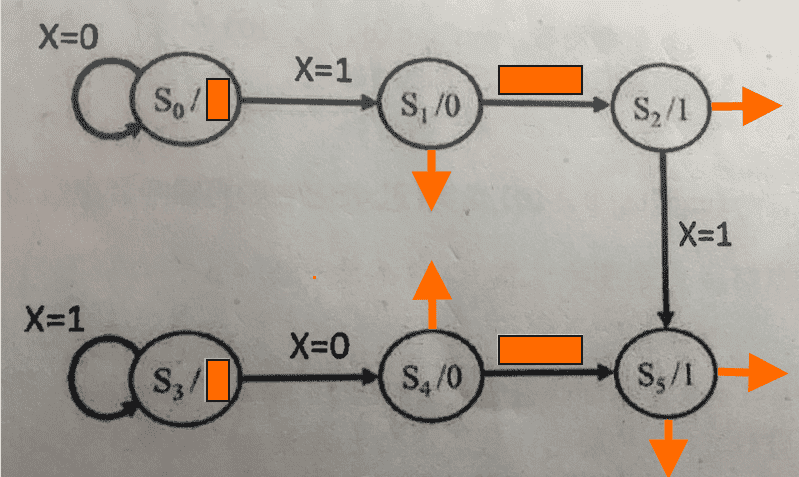#### Attachments

It is like a puzzle. As you have stated, it is an incomplete state transition diagram. You need to complete it in such a way that it performs the task of "observes the time series of input X and outputs 1 when time series 010 or 101 appears, and 0 otherwise". The highlighted areas below need your attention.
View attachment 226281
i don't understand, is it always start from S0?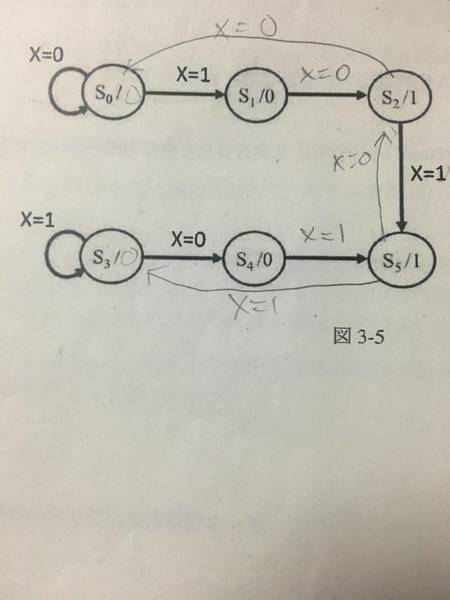do i need to pay attention to input x only?
is it always start from S0? i know x is input and S is flipflop and S0/.. is output.
such as S0->S1->S2 = 0->1->0 , so output S2/1 ?
then from S2 , i need to go to S3?

#### Attachments

It is like a puzzle. As you have stated, it is an incomplete state transition diagram. You need to complete it in such a way that it performs the task of "observes the time series of input X and outputs 1 when time series 010 or 101 appears, and 0 otherwise". The highlighted areas below need your attention.
View attachment 226281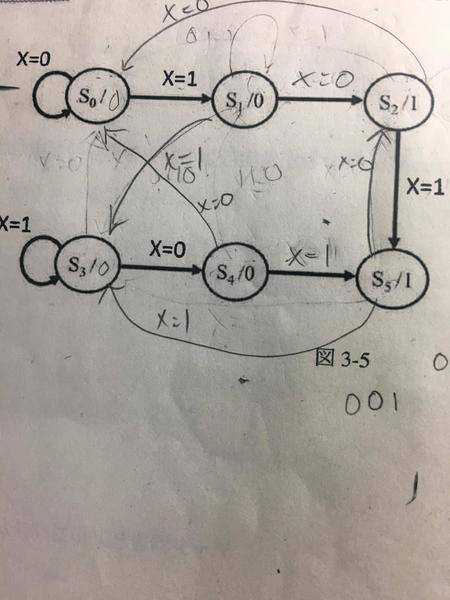after some youtube tutorial,is it correct?

#### Attachments

Yes, it is correct. Good work by you! For this kind of work, you may want to find a better eraser!

Yes, it is correct. Good work by you! For this kind of work, you may want to find a better eraser!
thanks for the help! :D do you read books to learn this?

let me re-write that:
The focus is on what input values are at the AND gate--for the clock pulse you care about. That is, the gate that directly outputs y. I was just trying emphasize lewando's point another way. The value of the output y can change with each clock pulse, right? So what determines that output value? The only thing that matters at that moment are the 2 inputs to the AND gate that feeds y.

I think you seem to be getting stuck on the instantaneous value of x or x'. But what I'm trying to say is, that really doesn't matter, because the circuit processes things sequentially. That's like when you're eating breakfast, trying to worry about what you are going to have for lunch. [Or maybe a better way of saying it: suppose you get packages every hour. At 8:00 you deal with the package you get at 8:00. There's another one on the way that will arrive at 9:00, but you can't do anything with it, because you don't know what it is yet.]

Until it's been clocked through, the present value of x is not relevant to the output.
thanks for the help! :D

do you read books to learn this?
Yes-- way before YouTube! I think I even still have them. Today, YouTube can be a great resource, because it simulates the lecture style of teaching. But it is a good idea to get at least a second source to verify or confirm the presented information.

Yes-- way before YouTube! I think I even still have them. Today, YouTube can be a great resource, because it simulates the lecture style of teaching. But it is a good idea to get at least a second source to verify or confirm the presented information.
but i mean learning from youtube will make me only understand how to solve this kind of problem, not the idea behind it, so when they change the problem, i will get lost again :/

So then look for resources that address the foundations and fundamentals of both analysis and synthesis. These are the books I have used:

The Art of Digital Design (Winkel, Prosser)
Digital Circuits and Microprocessors (Taub)

They are relatively affordable (used, from Amazon).

I'm sure there are plenty of on-line resources as well.

thanks for the help! :D
Sure...